Ch 2. Derivatives I Multimedia Engineering Math DerivativeFormulas TrigonometricFunctions Differentiability ChainRule ImplicitDifferentiation
 Chapter 1. Limits 2. Derivatives I 3. Derivatives II 4. Mean Value 5. Curve Sketching 6. Integrals 7. Inverse Functions 8. Integration Tech. 9. Integrate App. 10. Parametric Eqs. 11. Polar Coord. 12. Series Appendix Basic Math Units Search eBooks Dynamics Fluids Math Mechanics Statics Thermodynamics Author(s): Hengzhong Wen Chean Chin Ngo Meirong Huang Kurt Gramoll ©Kurt GramollMATHEMATICS - THEORY

The problems involving rate of changes exist in many area of engineering research. Since this type of limit happens so widely, it is given a special name - derivative.

Derivatives

The notation of derivative is f '(a). The derivative of a function f at a given point a is defined as: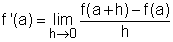(1)

The definition does assume that the limit exists. In order to extend this definition, let x = a + h, substitute x into f '(a) and will get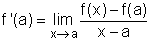(2)

The notation of a derivative can be written as: f '(a), y ', df/dx, dy/dx, Df(x), Dxf(x).

Explanation of the Derivative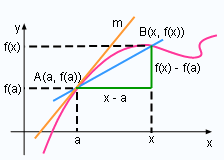Tangent

A good way to understand derivatives is to think about a tangent line. According to the definition of the tangent line to a curve y = f(x) at point A(a, f(a)), the tangent line can be written as: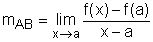(3)

Notice that this definition is the same as the definition of derivative f '(a). In other words, the tangent line to y = f(x) at point A(a, f(a)) is the line that passes through (a, f(a)) and whose slop is equal to the derivative of f at a.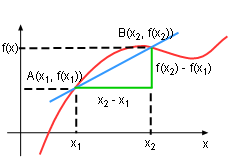Rate of Change

In a previous section, the concept of rate of change was introduced. Is it related to derivative? The answer is yes.

In a small interval [x1, x2], the changes in x is

Δx = x2 - x1

The corresponding change in y is

Δy = y2 - y1

The instantaneous rate of change isAccording to equation (3), r is the derivative of f(x) at x1

Derivative Formulas
Calculating derivative according to its definition is tedious. Some rules have been developed for finding derivatives without having to use the definition directly.
 F(x) F '(x) c 0 xn nxn-1 cf(x) cf '(x) f(x) + g(x) f '(x) + g '(x) f(x) - g(x) f '(x) - g '(x) f(x)g(x) f '(x)g(x) + f (x)g '(x) f(x)/g(x) (f '(x)g(x) - f (x)g '(x))/g2(x) x-n -nx-n-1

• If F is a constant function, F(x) = c,
then F '(x) = 0.
• If F(x) = xn, where n is a positive integer,
then F '(x) = nxn-1.
• Assume that c is constant and f '(x) and g '(x) exist.
• If F(x) = cf(x), then F '(x) = cf '(x)
• If F(x) = f(x) + g(x), then F '(x) = f '(x) + g '(x)
• If F(x) = f(x) - g(x), then F '(x) = f '(x) - g '(x)
• If F(x) = f(x)g(x), then
F '(x) = f '(x)g(x) + f (x)g '(x)
• If F(x) = f(x)/g(x), then
F '(x) = (f '(x)g(x) - f (x)g '(x))/g2(x)
• If F(x) = x-n, where n is a positive integer, then
F '(x) = -nx-n-1